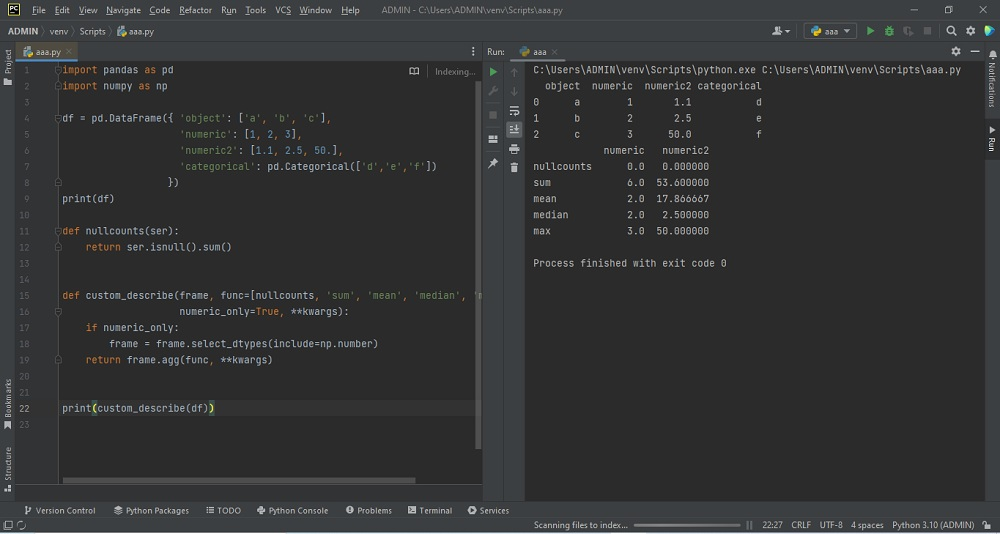# Pandas Data Frame Summary Tableby vsasikalabeUpdated: Mar 1, 2023Solution Kit

NumPy is a most familiar library in Python. It has many functions for doing tasks with the one-dimensional or multi-dimensional array. Some of the useful functions are mean(), min(), max(), average(), sum(), median(), etc.

To return the arithmetic mean value of the array elements, the mean() function is used. The arithmetic mean is estimated by dividing the sum of all array data by the total number present in the array elements. It will calculate the mean value of the particular axis if the particular axis is mentioned in the function.max() function is used for finding the maximum value from the array elements or the elements of the particular array axis. The min() function determines the minimum value from the array elements or the particular array axis.

We have to create a data frame, as mentioned in the snippet. The Describe function returns the statistical summary of the data frame or series. This includes the columns' null counts, sum, mean, median, and min-max values. The agg() method will allow you to apply a function in an array. It is also used to apply a function to be executed along one of the axes of the Data Frame. By default, 0, which is the index (row) axis. The agg() method is known as the aggregate() method.

• Sum-Total Value
• Mean – Average value of given values
• Median – Middle value
• Max-Maximum value in the series.

Here is an example of how to create a Pandas Data Frame summary table:Preview of the output that you will get on running this code from your IDE.

### Code

In this solution we used pandas and numpy library of python.

### Instructions

Follow the steps carefully to get the output easily.

2. Install pandas on your IDE from python interpreter in setting options.
3. Create new python file on your IDE.
4. Copy the snippet using the 'copy' button and paste (line no 1 to 22) it in your python file.
5. import the pandas and numpy library.Add print (custom_describe(df)) to the end.
6. Run the current file to generate the output.

I hope you found this useful. I have added the link to dependent library, version information in the following sections.

I found this code snippet by searching for ' Pandas Data Frame Summary Table ' in kandi. You can try any such use case!

### Environment Tested

I tested this solution in the following versions. Be mindful of changes when working with other versions.

1. PyCharm Community Edition 2022.3.1
2. The solution is created in Python 3.11.1 Version
3. pandas 1.5.2 Version
4. numpy 1.24.1 version

Using this solution, we can do Pandas Data Frame Summary Table in python.This process also facilities an easy to use, hassle free method to create a hands-on working version of code in python which would help us to do Pandas Data Frame Summary Table.

### Dependent Libraries

pandasby pandas-dev

Python37360Version:v2.0.0rc1Flexible and powerful data analysis / manipulation library for Python, providing labeled data structures similar to R data.frame objects, statistical functions, and much more

Support
Quality
Security
Reuse

pandasby pandas-dev

Python37360Version:v2.0.0rc1License: Permissive (BSD-3-Clause)

Flexible and powerful data analysis / manipulation library for Python, providing labeled data structures similar to R data.frame objects, statistical functions, and much more
Support
Quality
Security
Reuse

numpyby numpy

Python22989Version:v1.24.2The fundamental package for scientific computing with Python.

Support
Quality
Security
Reuse

numpyby numpy

Python22989Version:v1.24.2License: Permissive (BSD-3-Clause)

The fundamental package for scientific computing with Python.
Support
Quality
Security